### Example 6.3 Two-Person Zero-Sum Game

Consider a two-person zero-sum game (where one person wins what the other person loses). The players make moves simultaneously, and each has a choice of actions. There is a payoff matrix that indicates the amount one player gives to the other under each combination of actions: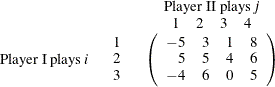If player I makes move i and player II makes move j, then player I wins (and player II loses)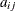. What is the best strategy for the two players to adopt? This example is simple enough to be analyzed from observation. Suppose player I plays 1 or 3; the best response of player II is to play 1. In both cases, player I loses and player II wins. So the best action for player I is to play 2. In this case, the best response for player II is to play 3, which minimizes the loss. In this case, (2, 3) is a pure-strategy Nash equilibrium in this game.

For illustration, consider the following mixed strategy case. Assume that player I selects i with probability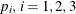, and player II selects j with probability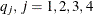. Consider player II’s problem of minimizing the maximum expected payout: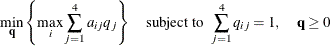This is equivalent to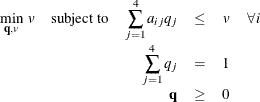The problem can be transformed into a more standard format by making a simple change of variables: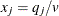. The preceding LP formulation now becomes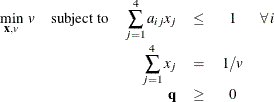which is equivalent to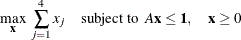where A is the payoff matrix andis a vector of 1’s. It turns out that the corresponding optimization problem from player I’s perspective can be obtained by solving the dual problem, which can be written as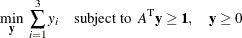You can model the problem and solve it by using PROC OPTMODEL as follows:

proc optmodel;
num a{1..3, 1..4}=[-5 3 1 8
5 5 4 6
-4 6 0 5];
var x{1..4} >= 0;
max f = sum{i in 1..4}x[i];
con c{i in 1..3}: sum{j in 1..4}a[i,j]*x[j] <= 1;
solve with lp / algorithm = ps presolver = none logfreq = 1;
print x;
print c.dual;
quit;


The optimal solution is displayed in Output 6.3.1.

Output 6.3.1: Optimal Solutions to the Two-Person Zero-Sum Game

The OPTMODEL Procedure

Problem Summary
Objective Sense Maximization
Objective Function f
Objective Type Linear

Number of Variables 4
Bounded Above 0
Bounded Below 4
Bounded Below and Above 0
Free 0
Fixed 0

Number of Constraints 3
Linear LE (<=) 3
Linear EQ (=) 0
Linear GE (>=) 0
Linear Range 0

Constraint Coefficients 11

Performance Information
Execution Mode Single-Machine
Number of Threads 1

Solution Summary
Solver LP
Algorithm Primal Simplex
Objective Function f
Solution Status Optimal
Objective Value 0.25

Primal Infeasibility 0
Dual Infeasibility 0
Bound Infeasibility 0

Iterations 4
Presolve Time 0.00
Solution Time 0.05

 x
1 0.00
2 0.00
3 0.25
4 0.00

 c.DUAL
1 0.00
2 0.25
3 0.00

The optimal solution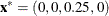with an optimal value of 0.25. Therefore the optimal strategy for player II is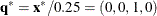. You can check the optimal solution of the dual problem by using the constraint suffix .dual. So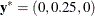and player I’s optimal strategy is (0, 1, 0). The solution is consistent with our intuition from observation.